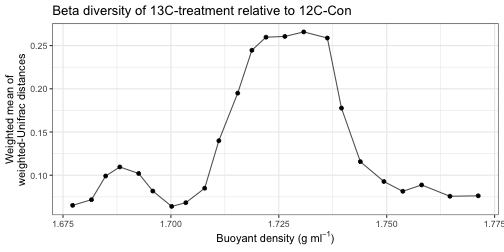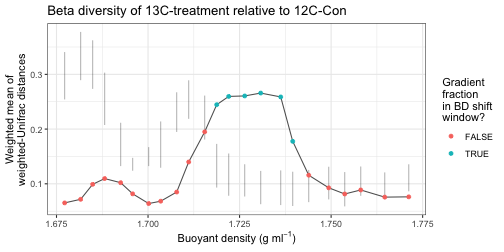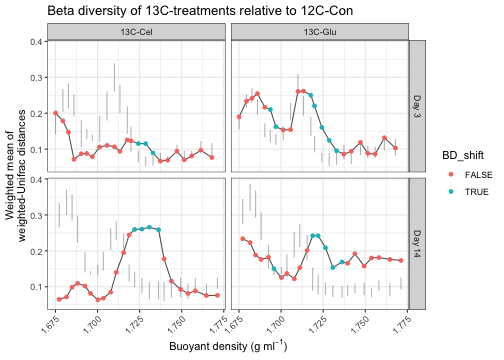# Making BD shift plots

Note: if you haven't checked out the beta diversity ordinations vignette yet, I recommend looking at that one first.

## Introduction

While the beta diversity ordinations of gradient fractions provides a nice overview of isotope incorporation at the whole-community level, it doesn't provide a good idea of the magnitude of this BD shift (ie., was there a lot of isotope incorporation or a little for each labeled-treatment?).

Let's assume that the gradient fraction communities of a labeled-treatment and its corresponding unlabled-control would be (approximately) the same at the same buoyant densities if no incorperation occured. If so, then the pairwise beta diversity between gradient fractions of the treatment vs control would (e.g., the beta diversity between the 13C & 12C communities at a BD of 1.75 g/ml1) would be ~0 (no differentiation) across the BD range. However, if some taxa incorporated isotope in the labeled-treatment, then they would shift to heavier buoyant densities, which would change the labeled-communities at the buoyant densities where the taxa used to be if unlabeled and the buoyant densities where the taxa have shifted to due to isotope incorporation.

In other words, if we make pairwise treatment-vs-control beta diversity calculations between gradient fraction communities, then we should see evidence of community-level BD shifts in the form of 'spikes' in beta diversity.

The only major issue with this approach is that the BD range of each gradient fraction varies from gradient to gradient. So, gradient fractions between gradients usually only partially overlap. To deal with this issue, we have taken the approach of weighting the beta diversity based on gradient fraction overlap. For instance, if 2 labeled-treatment fractions overlapped 1 control fraction by 40% and 60%, then the final beta diversity value would be the weighted average of treatment fraction 1 (40% weight) and treatment fraction 2 (60% weight). Note that this makes all beta diversity values (and their associated buoyant densities) relative to the control.

The following analysis measures these community-wide BD shifts with the following:

1. Splitting the dataset into pairwise comparisons between each labeled-treatment and its corresponding unlabeled control.
2. The percent BD overlap of treatment gradient fractions relative to the control are calculated.
3. For overlapping gradient fractions in each treatment-control comparison, beta diversity is calculated between the gradient fraction communities.
4. The weighted mean beta diversity (weighted by % fraction overlap) is calculated.
5. The resulting data.frame can then easily plotted with ggplot.

Moreover, a permutation test is conducted to identify “BD shift windows”, which are regions of high beta-diversity that likley resulted from BD shifts of taxa in the treatment (and not in the unlabeled control). The method involves permuting OTU abundances (HTSSIP offers multiple permutation methods; see `BD_shift()`), an re-calculating weighted beta-diversity values among overlapping fractions in the treatment versus the control.

## Dataset

First, let's load some packages including `HTSSIP`.

``````library(dplyr)
library(tidyr)
library(ggplot2)
library(HTSSIP)
``````

Also let's get an overview of the phyloseq object that we're going to use.

``````physeq_S2D2
``````
``````## phyloseq-class experiment-level object
## otu_table()   OTU Table:         [ 1072 taxa and 139 samples ]
## sample_data() Sample Data:       [ 139 samples by 17 sample variables ]
## tax_table()   Taxonomy Table:    [ 1072 taxa by 8 taxonomic ranks ]
## phy_tree()    Phylogenetic Tree: [ 1072 tips and 1071 internal nodes ]
``````

## Subsetting the phyloseq object

As with the beta diversity ordinations, we are going to split up the dataset into individual labeled-treamtent + corresponding unlabeled-control comparisons. Treatment-control correspondence is based on the day from substrate addition. So, we have to parse the dataset by Substrate & Day.

``````params = get_treatment_params(physeq_S2D2, c('Substrate', 'Day'))
params = dplyr::filter(params, Substrate!='12C-Con')
ex = "(Substrate=='12C-Con' & Day=='\${Day}') | (Substrate=='\${Substrate}' & Day == '\${Day}')"
physeq_S2D2_l = phyloseq_subset(physeq_S2D2, params, ex)
physeq_S2D2_l
``````
``````## \$`(Substrate=='12C-Con' & Day=='3') | (Substrate=='13C-Cel' & Day == '3')`
## phyloseq-class experiment-level object
## otu_table()   OTU Table:         [ 1072 taxa and 46 samples ]
## sample_data() Sample Data:       [ 46 samples by 17 sample variables ]
## tax_table()   Taxonomy Table:    [ 1072 taxa by 8 taxonomic ranks ]
## phy_tree()    Phylogenetic Tree: [ 1072 tips and 1071 internal nodes ]
##
## \$`(Substrate=='12C-Con' & Day=='14') | (Substrate=='13C-Cel' & Day == '14')`
## phyloseq-class experiment-level object
## otu_table()   OTU Table:         [ 1072 taxa and 46 samples ]
## sample_data() Sample Data:       [ 46 samples by 17 sample variables ]
## tax_table()   Taxonomy Table:    [ 1072 taxa by 8 taxonomic ranks ]
## phy_tree()    Phylogenetic Tree: [ 1072 tips and 1071 internal nodes ]
##
## \$`(Substrate=='12C-Con' & Day=='14') | (Substrate=='13C-Glu' & Day == '14')`
## phyloseq-class experiment-level object
## otu_table()   OTU Table:         [ 1072 taxa and 47 samples ]
## sample_data() Sample Data:       [ 47 samples by 17 sample variables ]
## tax_table()   Taxonomy Table:    [ 1072 taxa by 8 taxonomic ranks ]
## phy_tree()    Phylogenetic Tree: [ 1072 tips and 1071 internal nodes ]
##
## \$`(Substrate=='12C-Con' & Day=='3') | (Substrate=='13C-Glu' & Day == '3')`
## phyloseq-class experiment-level object
## otu_table()   OTU Table:         [ 1072 taxa and 46 samples ]
## sample_data() Sample Data:       [ 46 samples by 17 sample variables ]
## tax_table()   Taxonomy Table:    [ 1072 taxa by 8 taxonomic ranks ]
## phy_tree()    Phylogenetic Tree: [ 1072 tips and 1071 internal nodes ]
``````

## Calculating BD shift

Now, let's just measure BD shift for just 1 subset (1 item in the list of phyloseq objects).

Note: we are just going to use 10 permutations to speed up the analysis.

``````wmean1 = BD_shift(physeq_S2D2_l[], nperm=10)
cat('Subset:', names(physeq_S2D2_l), '\n')
``````
``````## Subset: (Substrate=='12C-Con' & Day=='14') | (Substrate=='13C-Cel' & Day == '14')
``````
``````wmean1 %>% head(n=3)
``````
``````##   perm_id           sample.x  distance Replicate.x IS__CONTROL.x BD_min.x
## 1       0 12C-Con.D14.R1_F12 0.2587625           1          TRUE 1.736237
## 2       0 12C-Con.D14.R1_F13 0.2553421           1          TRUE 1.730773
## 3       0 12C-Con.D14.R1_F23 0.1449341           1          TRUE 1.692527
##   BD_max.x BD_range.x perc_overlap n_over_fracs wmean_dist
## 1 1.739516   0.003279    100.00000            1  0.2587625
## 2 1.736237   0.005464     19.98536            2  0.2658108
## 3 1.695805   0.003278     33.34350            2  0.1020456
##   wmean_dist_CI_low_global wmean_dist_CI_high_global wmean_dist_CI_low
## 1               0.07081277                 0.3327846        0.06112415
## 2               0.07081277                 0.3327846        0.06271802
## 3               0.07081277                 0.3327846        0.13232953
##   wmean_dist_CI_high
## 1          0.1245348
## 2          0.1236211
## 3          0.2116010
``````

Note that the `sample.x` column is all 12C-Con control samples, while the comparison column (`sample.y`) is the treatment gradient fraction samples. The “wmeandist_CI[low/high]” columns list the CI intervals (calculated by the permutation test). The “wmeandist_CI*global” columns define the CI interval for all gradient fractions.

OK. Let's plot the results!

``````x_lab = bquote('Buoyant density (g '* ml^-1*')')
y_lab = 'Weighted mean of\nweighted-Unifrac distances'
ggplot(wmean1, aes(BD_min.x, wmean_dist)) +
geom_line(alpha=0.7) +
geom_point() +
labs(x=x_lab, y=y_lab, title='Beta diversity of 13C-treatment relative to 12C-Con') +
theme_bw()
``````Each point represents the weighted mean of beta diversity values between all 13C-treatment fractions that overlap a particular 12C-control fraction, so there should be 1 point per 12C-control gradient fraction.

Note the 2 spikes in beta diversity. The 2nd spike is larger than the first, which is likely due to more taxa at the 'light' gradient fractions (1st spike), so a loss of a few taxa (due to BD shifting) impacts beta diveristy less than at 'heavy' gradient fractions, where there's less taxa.

### Identifying BD shift windows

Let's identify the BD shift windows. “BD shift” fractions are those greater than the bootstrap CI. To reduce potential noice, I'm going to define BD shift windows as 3 consecutive “BD shift” fractions.

``````wmean1_m = wmean1 %>%
mutate(BD_shift = wmean_dist > wmean_dist_CI_high) %>%
arrange(BD_min.x) %>%
mutate(window = (BD_shift == TRUE & lag(BD_shift) == TRUE & lag(BD_shift, 2) == TRUE) |
(BD_shift == TRUE & lag(BD_shift) == TRUE & lead(BD_shift) == TRUE) |
(BD_shift == TRUE & lead(BD_shift) == TRUE & lead(BD_shift, 2) == TRUE),
BD_shift = BD_shift == TRUE & window == TRUE,
BD_shift = ifelse(is.na(BD_shift), FALSE, BD_shift))

``````
``````##   perm_id           sample.x   distance Replicate.x IS__CONTROL.x BD_min.x
## 1       0 12C-Con.D14.R1_F27 0.06874209           1          TRUE 1.677228
## 2       0 12C-Con.D14.R1_F26 0.09574961           1          TRUE 1.681599
## 3       0 12C-Con.D14.R1_F25 0.15970518           1          TRUE 1.684878
##   BD_max.x BD_range.x perc_overlap n_over_fracs wmean_dist
## 1 1.681599   0.004371     24.98284            2 0.06516101
## 2 1.684878   0.003279     33.33333            2 0.07170448
## 3 1.688156   0.003278     33.34350            2 0.09908418
##   wmean_dist_CI_low_global wmean_dist_CI_high_global wmean_dist_CI_low
## 1               0.07081277                 0.3327846         0.2541746
## 2               0.07081277                 0.3327846         0.2893559
## 3               0.07081277                 0.3327846         0.2735027
##   wmean_dist_CI_high BD_shift window
## 1          0.3407819    FALSE  FALSE
## 2          0.3775667    FALSE  FALSE
## 3          0.3622643    FALSE  FALSE
``````
``````x_lab = bquote('Buoyant density (g '* ml^-1*')')
y_lab = 'Weighted mean of\nweighted-Unifrac distances'
ggplot(wmean1_m, aes(BD_min.x, wmean_dist)) +
geom_line(alpha=0.7) +
geom_linerange(aes(ymin=wmean_dist_CI_low,
ymax=wmean_dist_CI_high),
alpha=0.3) +
geom_point(aes(color=BD_shift)) +
scale_color_discrete('Gradient\nfraction\nin BD shift\nwindow?') +
labs(x=x_lab, y=y_lab, title='Beta diversity of 13C-treatment relative to 12C-Con') +
theme_bw()
``````The line ranges represent the bootstrap CIs. This permutation test helps to non-subjectively identify BD shift windows, where beta-diversity is higher than expected under the null model.

Note: more permutations should be used for real analyses.

## Calculating BD shift for all treatments

Now let's run `BD_shift()` on all phyloseq objects in our list. We'll use `plyr::ldply()` for this because it preserves the list names in the resulting data.frame (list names are assigned to `.id` by default).

``````wmean = plyr::ldply(physeq_S2D2_l, BD_shift, nperm=5)
``````
``````##                                                                       .id
## 1 (Substrate=='12C-Con' & Day=='3') | (Substrate=='13C-Cel' & Day == '3')
## 2 (Substrate=='12C-Con' & Day=='3') | (Substrate=='13C-Cel' & Day == '3')
## 3 (Substrate=='12C-Con' & Day=='3') | (Substrate=='13C-Cel' & Day == '3')
##   perm_id          sample.x   distance Replicate.x IS__CONTROL.x BD_min.x
## 1       0 12C-Con.D3.R3_F19 0.07506826           1          TRUE 1.705640
## 2       0 12C-Con.D3.R3_F13 0.11524238           1          TRUE 1.728588
## 3       0 12C-Con.D3.R3_F14 0.12153372           1          TRUE 1.724217
##   BD_max.x BD_range.x perc_overlap n_over_fracs wmean_dist
## 1 1.710011   0.004371     12.49142            2  0.1105012
## 2 1.732959   0.004371    100.00000            1  0.1152424
## 3 1.728588   0.004371     74.99428            2  0.1153831
##   wmean_dist_CI_low_global wmean_dist_CI_high_global wmean_dist_CI_low
## 1               0.06396301                 0.2749192        0.17970105
## 2               0.06396301                 0.2749192        0.05233188
## 3               0.06396301                 0.2749192        0.06334878
##   wmean_dist_CI_high
## 1         0.26101204
## 2         0.07928588
## 3         0.09538421
``````

Alright, let's plot the data!

``````# formatting the treatment names to look a bit better as facet labels
wmean = wmean %>%
mutate(Substrate = gsub('.+(13C-[A-z]+).+', '\\1', .id),
Day = gsub('.+Day ==[ \']*([0-9]+).+', 'Day \\1', .id),
Day = Day %>% reorder(gsub('Day ', '', Day) %>% as.numeric))

# calculating BD shift windows
wmean = wmean %>%
mutate(BD_shift = wmean_dist > wmean_dist_CI_high) %>%
arrange(Substrate, BD_min.x) %>%
group_by(Substrate) %>%
mutate(window = (BD_shift == TRUE & lag(BD_shift) == TRUE & lag(BD_shift, 2) == TRUE) |
(BD_shift == TRUE & lag(BD_shift) == TRUE & lead(BD_shift) == TRUE) |
(BD_shift == TRUE & lead(BD_shift) == TRUE & lead(BD_shift, 2) == TRUE),
BD_shift = BD_shift == TRUE & window == TRUE,
BD_shift = ifelse(is.na(BD_shift), FALSE, BD_shift)) %>%
ungroup()

# plotting, with facetting by 13C-treatment
ggplot(wmean, aes(BD_min.x, wmean_dist)) +
geom_line(alpha=0.7) +
geom_linerange(aes(ymin=wmean_dist_CI_low,
ymax=wmean_dist_CI_high),
alpha=0.3) +
geom_point(aes(color=BD_shift)) +
labs(x=x_lab, y=y_lab,
title='Beta diversity of 13C-treatments relative to 12C-Con') +
facet_grid(Day ~ Substrate) +
theme_bw() +
theme(axis.text.x = element_text(angle=45, hjust=1))
``````As you can see, the 'heavy' beta diversity spike is stronger for 13C-Glucose at Day 3 versus 13C-Cellulose, but this pattern reverses at Day 14 of the substrate incubation. These results are to be expected, given that glucose is more labile than cellulose.

# Session info

``````sessionInfo()
``````
``````## R version 3.4.3 (2017-11-30)
## Platform: x86_64-apple-darwin15.6.0 (64-bit)
## Running under: macOS Sierra 10.12.6
##
## Matrix products: default
## BLAS: /Library/Frameworks/R.framework/Versions/3.4/Resources/lib/libRblas.0.dylib
## LAPACK: /Library/Frameworks/R.framework/Versions/3.4/Resources/lib/libRlapack.dylib
##
## locale:
##  C/en_US.UTF-8/en_US.UTF-8/C/en_US.UTF-8/en_US.UTF-8
##
## attached base packages:
##  stats     graphics  grDevices utils     datasets  methods   base
##
## other attached packages:
##  phyloseq_1.22.3 HTSSIP_1.4.1    ggplot2_3.2.0   tidyr_0.8.3
##  dplyr_0.8.0.1
##
## loaded via a namespace (and not attached):
##   tidyselect_0.2.5    reshape2_1.4.3      purrr_0.3.2
##   splines_3.4.3       lattice_0.20-38     rhdf5_2.22.0
##   colorspace_1.4-1    stats4_3.4.3        mgcv_1.8-28
##  survival_2.44-1.1   rlang_0.4.0         pillar_1.4.1
##  glue_1.3.1          withr_2.1.2         BiocGenerics_0.24.0
##  foreach_1.4.4       plyr_1.8.4          stringr_1.4.0
##  zlibbioc_1.24.0     Biostrings_2.46.0   munsell_0.5.0
##  gtable_0.3.0        codetools_0.2-16    evaluate_0.14
##  labeling_0.3        Biobase_2.38.0      knitr_1.18
##  permute_0.9-5       IRanges_2.12.0      biomformat_1.6.0
##  parallel_3.4.3      highr_0.8           Rcpp_1.0.1
##  scales_1.0.0        vegan_2.5-1         S4Vectors_0.16.0
##  jsonlite_1.6        XVector_0.18.0      digest_0.6.19
##  stringi_1.4.3       grid_3.4.3          ade4_1.7-13
##  tools_3.4.3         magrittr_1.5        lazyeval_0.2.2
##  tibble_2.1.1        cluster_2.0.6       crayon_1.3.4
##  ape_5.3             pkgconfig_2.0.2     MASS_7.3-51.4
##  Matrix_1.2-17       data.table_1.10.4-3 assertthat_0.2.1
##  iterators_1.0.10    R6_2.4.0            multtest_2.34.0
##  igraph_1.2.4        nlme_3.1-131        compiler_3.4.3
``````